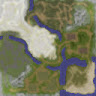## The Traveling Salesman Problem Using Simulated Annealing In C#

The Traveling Salesman Problem (TSP) is a standard combinatorial optimization problem. The goal is to find the best route that visits all cities, where best route usually means shortest distance.

Simulated Annealing is a very simple heuristic for solving combinatorial optimization problems. Briefly:

```make an initial guess route
loop
generate a new route
if new route is better, accept route
if new route is worse, accept
route anyway with a small probability
decrease accept_probability slightly
end-loop
return best route found
```

The devil is in the details: defining how to generate a new route and modifying the small acceptance probability of accepting a worse route.

I implemented simulated annealing for TSP using the Python language for an article in Visual Studio Magazine. Here is a C# implementation of the VSM article program.The C# program results (top) are slightly different than the Python program results (bottom) because simulated annealing is probabilistic and the C# and Python random number generation functions are a bit different.

Because Python and C# are both C-family languages, it was relatively easy to refactor the original Python code to C# code. I did have to implement a Shuffle() function in C# to replace the built-in Python shuffle() function, but I’ve done that many times (using the Fisher-Yates mini-algorithm).

Good fun.When I was just out of college, I worked on a cruise ship for a couple of years. I got to visit dozens of interesting countries — but all of them bordered the ocean. I know next to nothing about land-locked places such as these three adjacent Central Asian countries. Left: Kazakhstan (population 19 million). Center: Uzbekistan (population 34 million). Right: Kyrgyzstan (population 7 million). I wonder how similar their spoken and written languages are.

Demo C# code. Replace “lt” and “gt” with symbols — my blogging editor chokes on these symbols.

```using System;

namespace TSP_Annealing
{
class TSP_Annealing_Program
{
static void Main(string[] args)
{
Console.WriteLine("\nBegin TSP simulated annealing C# ");

int nCities = 20;
Console.WriteLine("\nSetting num_cities = " + nCities);
Console.WriteLine("\nOptimal solution is 0, 1, 2, . . " +
(nCities - 1));
Console.WriteLine("Optimal soln has total distance = " +
(nCities - 1));
Random rnd = new Random(2);
int maxIter = 25000;
double startTemperature = 10000.0;
double alpha = 0.98;

Console.WriteLine("\nSettings: ");
Console.WriteLine("max_iter = " + maxIter);
Console.WriteLine("start_temperature = " +
startTemperature.ToString("F1"));
Console.WriteLine("alpha = " + alpha);

Console.WriteLine("\nStarting solve() ");
int[] soln = Solve(nCities, rnd, maxIter,
startTemperature, alpha);
Console.WriteLine("Finished solve() ");

Console.WriteLine("\nBest solution found: ");
ShowArray(soln);
double dist = TotalDist(soln);
Console.WriteLine("\nTotal distance = " +
dist.ToString("F1"));

Console.WriteLine("\nEnd demo ");
}  // Main()

static double TotalDist(int[] route)
{
double d = 0.0;  // total distance between cities
int n = route.Length;
for (int i = 0; i "lt" n - 1; ++i)
{
if (route[i] "lt" route[i + 1])
d += (route[i + 1] - route[i]) * 1.0;
else
d += (route[i] - route[i + 1]) * 1.5;
}
return d;
}

static double Error(int[] route)
{
int n = route.Length;
double d = TotalDist(route);
double minDist = n - 1;
return d - minDist;
}

static int[] Adjacent(int[] route, Random rnd)
{
int n = route.Length;
int[] result = (int[])route.Clone();  // shallow is OK
int i = rnd.Next(0, n); int j = rnd.Next(0, n);
int tmp = result[i];
result[i] = result[j]; result[j] = tmp;
return result;
}

static void Shuffle(int[] route, Random rnd)
{
// Fisher-Yates algorithm
int n = route.Length;
for (int i = 0; i "lt" n; ++i)
{
int rIndx = rnd.Next(i, n);
int tmp = route[rIndx];
route[rIndx] = route[i];
route[i] = tmp;
}
}

static void ShowArray(int[] arr)
{
int n = arr.Length;
Console.Write("[ ");
for (int i = 0; i "lt" n; ++i)
Console.WriteLine(" ]");
}

static int[] Solve(int nCities, Random rnd, int maxIter,
double startTemperature, double alpha)
{
double currTemperature = startTemperature;
int[] soln = new int[nCities];
for (int i = 0; i "lt" nCities; ++i) { soln[i] = i; }
Shuffle(soln, rnd);
Console.WriteLine("Initial guess: ");
ShowArray(soln);

double err = Error(soln);
int iteration = 0;
while (iteration "lt" maxIter && err "gt" 0.0)
{
if (adjErr "lt" err)  // better route
{
}
else
{
double acceptProb =
double p = rnd.NextDouble(); // corrected
if (p "lt" acceptProb)  // accept anyway
{
}
}

if (currTemperature "lt" 0.00001)
currTemperature = 0.00001;
else
currTemperature *= alpha;
++iteration;
}  // while

return soln;
}  // Solve()

}  // Program class
}  // ns
```
This entry was posted in Machine Learning. Bookmark the permalink.

### 4 Responses to The Traveling Salesman Problem Using Simulated Annealing In C#

1.John Rogers says:

Towards the end:
double p = rnd.Next();
Should be:
double p = rnd.NextDouble();

2.jamesdmccaffrey says:

Ack! You are absolutely correct. I fixed the code in the post. Mahalo!

3.Frank Holiday says:

Hi James,
I have a few questions, the main one is if I have say up to 50 rooms and i wanted to order them by a sort of travelling salesman shortest path using simulated annealing would I need to create a matrix of say the distances between each 2d coordinate point via a pythagorean hypoteneuse distance and then could i simply modify some of the methods like total distance? Also I did a test of your code in vs2022 .net frameworks 4.7.2 c# console and had a score of 28.5, this seems to vary from all the other results. The order was simply the inverse of the optimal order of say 1,2,3, n+1.
Cheers Frank

•jamesdmccaffrey says:

Yes, in most non-demo cases you need a matrix of Euclidean distances. And yes, results can vary because of the different random number generators.